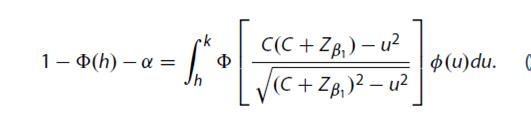## QUAD function evaluation for a complex objective function

Hi,

The following code finds the root of an objective function when the INTERVAL (see in red below) is chosen carefully to be {1,3} but fails to produce when the interval is chosen as {0 3} for example. The problem is that in order for function FUN1 to work (C+ZBETA1-U) has to be > 0; is there any way to specify a general interval like {-3,3} but the code can still work?

PROC IML;

START FUN1(U) GLOBAL(C,ZBETA1,H,K,ALPHA);

/*SELECT THE VALUE OF C SUCH THAT THE DENOMINATOR BECOMES POSITIVE*/;

IF (C+ZBETA1-U)>0 THEN DO;

ARG = (C*(C+ZBETA1)-U**2)/SQRT((C+ZBETA1)**2-U**2);

YY = CDF("NORMAL", ARG)*PDF("NORMAL", U);

END;

IF (C+ZBETA1-U)<=0 THEN RETURN(0);;

RETURN(YY);

FINISH;

START FUN2(PARAM) GLOBAL(C,ZBETA1,H,K,ALPHA);

C = PARAM;

K1 = K><(C+ZBETA1);

FF = 1 - CDF("NORMAL",H) - ALPHA - INT;

RETURN(FF);

FINISH;

H = 1;

K = 2.76;

ALPHA = 0.025;

BETA1 = 0.2;

ZBETA1 = QUANTILE("NORMAL",1-BETA1);

INTERVALS = {0,3}; /*THIS INTERVAL SHOULD BE SET SUCH THAT THE DENOMINATOR OF INTEGRAND REMAINS POSITIVE*/

Z = FROOT("FUN2", INTERVALS);

PRINT Z;

QUIT;

1 ACCEPTED SOLUTION

Accepted Solutions

## Re: QUAD function evaluation for a complex objective function

How do the limits of integration, H and K, depend on C? The integrand is defined only when  -(C+Z) < u < C+Z, so the integral does not make sense if H and K are outside of that interval.  In FUN1, you are trying to protect against negative square roots by declaring that FUN1=0 when the term under the square root is negative. This creates a point of discontinuity since lim u->C+Z from the left is -infinity, but from the right is zero (because of your artificial definition)..

I think I would try to determine valid values for H and K inside of FUN2 and avoid setting FUN1=0 when the integrand is undefined.

3 REPLIES 3

## Re: QUAD function evaluation for a complex objective function

What problem are you trying to solve?

## Re: QUAD function evaluation for a complex objective function

Hi Rick,

The basic problem is to find the value of C from the equation below where h, k, alpha are provided, capital/small phi are CDF/PDF of normal. The value of C is known to be 1.923 for beta1 = 0.2.## Re: QUAD function evaluation for a complex objective function

How do the limits of integration, H and K, depend on C? The integrand is defined only when  -(C+Z) < u < C+Z, so the integral does not make sense if H and K are outside of that interval.  In FUN1, you are trying to protect against negative square roots by declaring that FUN1=0 when the term under the square root is negative. This creates a point of discontinuity since lim u->C+Z from the left is -infinity, but from the right is zero (because of your artificial definition)..

I think I would try to determine valid values for H and K inside of FUN2 and avoid setting FUN1=0 when the integrand is undefined.

From The DO Loop